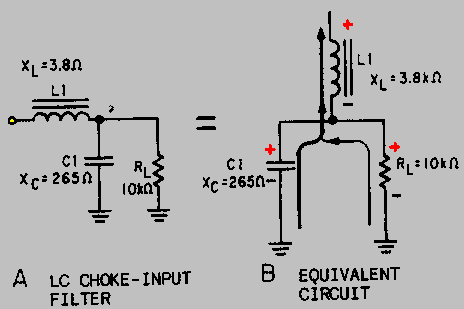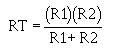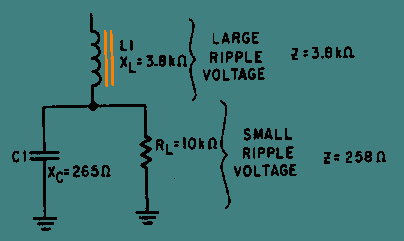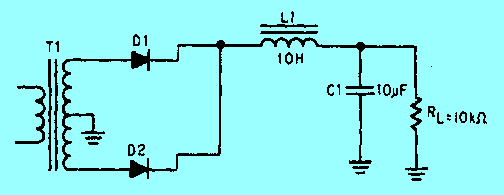Custom SearchLet's go a step further and redraw the filter circuit so that you can see the voltage divider action. Refer to view A of figure 4-24. Remember, the 165 volts peak 60 hertz provided by the rectifier consists of both an ac and a dc component. This first discussion will be about the ac component. From the figure, you see that the capacitor (C1) offers the least opposition (265 ohms) to the ac component. Therefore, the greater amount of ac will flow through C1. (The heavy line in view B indicates the ac current flow through the capacitor.) Thus the capacitor bypasses, or shunts, most of the ac around the load. Figure 4-24. - Ac component in an LC choke-input filter.By combining the XC of C1 and the resistance of RL into an equivalent circuit (view B), you will have an equivalent impedance of 265 ohms. As a formula;You now have a voltage divider as illustrated in figure 4-25. You should see that because of the impedance ratios, a large amount of ripple voltage is dropped across L1, and a substantially smaller amount is dropped across C1 and RL. You can further increase the ripple voltage across L1 by increasing the inductance (XL = 2pfL). Figure 4-25. - Equivalent circuit of an LC choke-input filter.Now let's discuss the dc component of the applied voltage. Remember, a capacitor offers an infinite impedance to the flow of direct current. The dc component, therefore, must flow through RL and L1. As far as the dc is concerned, the capacitor does not exist. The coil and the load are therefore in series with each other. The dc resistance of a filter choke is very low (50 ohms average). Consequently, most of the dc component is developed across the load and a very small amount of the dc voltage is dropped across the coil, as shown in figure 4-26. Figure 4-26. - Dc component in an LC choke-input filter.As you may have noticed, both the ac and the dc components flow through L1. Because it is frequency sensitive, the coil provides a large resistance to ac and a small resistance to dc. In other words, the coil opposes any change in current. This property makes the coil a highly desirable filter component. Note that the filtering action of the LC choke-input filter is improved when the filter is used in conjunction with a full-wave rectifier, as shown in figure 4-27. This is due to the decrease in the XC of the filter capacitor and the increase in the XL of the choke. Remember, ripple frequency of a full-wave rectifier is twice that of a half-wave rectifier. For 60-hertz input, the ripple will be 120 hertz. The XC of C1 and the XL of L1 are calculated as follows:Figure 4-27. - Full-wave rectifier with an LC choke-input filter.When the XC of a filter capacitor is decreased, it provides less opposition to the flow of ac. The greater the ac flow through the capacitor, the lower the flow through the load. Conversely, the larger the XL of the choke, the greater the amount of ac ripple developed across the choke; consequently, less ripple is developed across the load and better filtering is obtained. Q.21 In an LC choke-input filter, what prevents the rapid charging of the capacitor?Q.22 What is the range of values usually chosen for a choke?Q.23 If the impedance of the choke is increased, will the ripple amplitude increase or decrease?Integrated Publishing, Inc. - A (SDVOSB) Service Disabled Veteran Owned Small Business